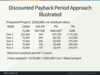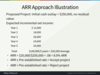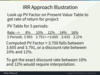Capital Budgeting: Evaluation Techniques Flashcards Preview

BEC > Capital Budgeting: Evaluation Techniques > Flashcards

Flashcards in Capital Budgeting: Evaluation Techniques Deck (52):
1

2

3

4

5

6

7

8

9

Discount Payback Period Illustrated:

Discount Payback Period Illustrated:10

11

12

13

14

15

ARR Approach Illustration:16

17

18

19

20

21

Net Present Value Approach: Illustration22

23

24

25

26

27

28

Question:Given a 10% discount rate with cash inflows of \$3,000 at the end of each year for five years and an initial investment of \$11,000, what is the net present value?A.  (\$9,500) B.  \$370 C.  \$4,000 D.  \$11,370

B.  \$370The net present value is \$370. The present value (PV) of expected cash inflows is determined by discounting those flows to their present value using the firm's discount rate (also called the hurdle rate). The difference between the resulting PV of cash inflows and the initial cost (which is at present value) is the net present value of the project. Cash inflows are \$3,000 at the end of each year for five years, which is an ordinary annuity. If (in the absence of a PV table of factors) you know the formula for the PV of an ordinary annuity it can be used. That would be:PVoa = C x [(1 - (1 / (1 + i)n)) / i]Where: C = Cash flow per year; i = interest rate; and n = number of years.Substituting: PV = \$3,000 x [(1 - (1/(1 + .10)5))/.10]; PV = \$3,000 x 3.79; PV of cash inflows = \$11,370 - initial investment \$11,000 = \$370 net present value.Even if you don't have PV factors or don't know the formula for PVoa, you can compute the answer (even easier than using the formula). For each year, discount the \$3,000 by the discount rate times the number of years, as follows:29

30

31

IRR Approach Illustration:

IRR Approach Illustration:Equation for NPV=0: Future annual cash inflows x PV factor = Investment Cost Rearranged Equation: PV factor = Investment Cost / Future annual cash inflows. Proposed Project: Initial cash outlay = \$37,500, no residual value; Expected CF= \$10,000 year for 5 yrs PV factor = Initial Cost/Expected Cash = \$37,500 / \$10,000 = 3.750 (PV factor) See attached:32

33

34

35

36

37

38

39

40

41

42

43

44

45

46

47

48

49

50

51

52